Preliminary results as of Winter 2014
(Moriond 2014 conference)

 The global CKM fit: Inputs and Numerical results The global CKM fit: Pulls The global CKM fit in the large (ρ-bar,η-bar) plane The global CKM fit in the small (ρ-bar,η-bar) plane (zoom) The global CKM fit in the large (ρs-bar,ηs-bar) plane Constraint from BR(B+→τ+ ν) The global CKM fit in the (|Vud|,|Vus) plane The global CKM fit in the (|Vcd|,|Vcs) plane Constraints on the angle γ/ϕ3 from B decays to charm

Numerical results:

 The results of the global CKM analysis include: Wolfenstein parameters, UT angles and sides, UTsangle and apex, CKM elements, theory parameters, rare branching fractions (B->lν, B->ll).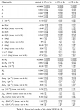Numerical Results

 Pulls for various inputs or parameters involved in the Standard Model global fit. Each pull (in units of σ) is computed by taking the square root of the difference between χ2min obtained including or not including direct information on the quantity. This corresponds to consider Δχ2X;min=χ2with data on X;min- χ2without data X;min as a random variable distributed with 1 degree of freedom, and reinterpret the probability of reaching the observed value in units of σ. The presence of a plateau in the Rfit model for theoretical uncertainties may lead to a vanishing pull for some quantities even in cases where the predicted and observed values are not identical. Many of the pulls presented in this plot are correlated [this is for instance the case for sin 2β and Br(B→τν)], so that the distribution of the corresponding p-values should not be expected to be flat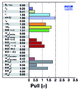eps png

Constraints in the (ρ-bar,η-bar) plane. The red hashed region of the global combination corresponds to 68% CL.
 α, β, γconvention ϕ1, ϕ2, ϕ3convention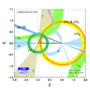eps png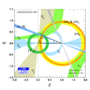eps png
Constraints in the (ρ-bar,η-bar) plane. The |Vub| constraint has been splitted in the two contributions: |Vub| from inclusive and exclusive semileptonic decays (plain dark green) and |Vub| from B+→τ+ ν (hashed green). The red hashed region of the global combination corresponds to 68% CL.
 α, β, γconvention ϕ1, ϕ2, ϕ3conventioneps png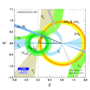eps png

The global CKM fit in the small (ρ-bar,η-bar) plane (zoom):

Zoomed constraints in the (ρ-bar,η-bar) plane.The red hashed region of the global combination corresponds to 68% CL.
 α, β, γconvention ϕ1, ϕ2, ϕ3convention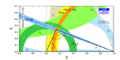eps png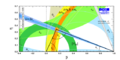eps png
Zoomed constraints in the (ρ-bar,η-bar) plane. The |Vub| constraint has been splitted in the two contributions: |Vub| from inclusive and exclusive semileptonic decays (plain dark green) and |Vub| from B+→τ+ ν (hashed green). The red hashed region of the global combination corresponds to 68% CL.
 α, β, γconvention ϕ1, ϕ2, ϕ3convention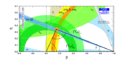eps png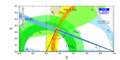eps png
Zoomed constraints in the (ρ-bar,η-bar) plane not including the angle measurements in the global fit.
 α, β, γconvention ϕ1, ϕ2, ϕ3convention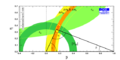eps png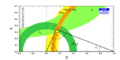eps png
Constraints in the (ρ-bar,η-bar) plane including only the angle measurements.
 α, β, γconvention ϕ1, ϕ2, ϕ3conventioneps png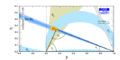eps png
Constraints from CP conserving quantities (|Vub / Vcb|, Δmd, (Δmd and Δms) and B+ →τ+ ν) in the (ρ-bar,η-bar) plane.
 α, β, γconvention ϕ1, ϕ2, ϕ3convention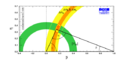eps pngeps png
Constraints from CP violating quantities (sin(2β), α, γ and εk) in the (ρ-bar,η-bar) plane.
 α, β, γconvention ϕ1, ϕ2, ϕ3convention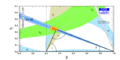eps png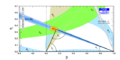eps png
Constraints from "Tree" quantities in the (ρ-bar,η-bar) plane (involving γ(DK) and α from the isospin analysis with the help of sin2β (charmonium), which gives another tree only γ measurement (the only assumption is that the ΔI=3/2 b-->d EW penguin amplitude is negligible)).
 α, β, γconvention ϕ1, ϕ2, ϕ3convention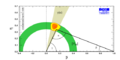eps png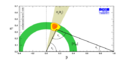eps png
Constraints from "Tree" quantities in the (ρ-bar,η-bar) plane (only γ(DK) is used).
 α, β, γconvention ϕ1, ϕ2, ϕ3convention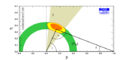eps png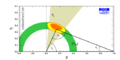eps png
Constraints from "Tree" quantities in the (ρ-bar,η-bar) plane with only input on |Vub| from semileptonic decays (ony γ(DK) is used).
 α, β, γconvention ϕ1, ϕ2, ϕ3convention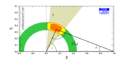eps png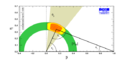eps png
Constraints from "Tree" quantities in the (ρ-bar,η-bar) plane with only input on |Vub| from exclusive semileptonic decays (only γ(DK) is used).
 α, β, γconvention ϕ1, ϕ2, ϕ3convention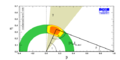eps png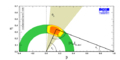eps png
Constraints from "Tree" quantities in the (ρ-bar,η-bar) plane with only input on |Vub| from inclusive semileptonic decays (only γ(DK) is used).
 α, β, γconvention ϕ1, ϕ2, ϕ3convention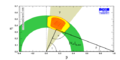eps png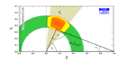eps png
Constraints from "Loop" quantities in the (ρ-bar,η-bar) plane.
 α, β, γconvention ϕ1, ϕ2, ϕ3convention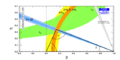eps png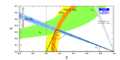eps png
Constraints in the (ρ-bar,η-bar) plane, not including the braching ratio of B+ → τ+ν in the global fit.
 α, β, γconvention ϕ1, ϕ2, ϕ3convention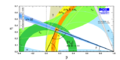eps png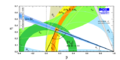eps png
Constraints in the (ρ-bar,η-bar) plane not including the measurement of sin2β in the global fit.
 α, β, γconvention ϕ1, ϕ2, ϕ3convention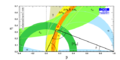eps png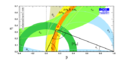eps png

Constraints in the (ρs-bar,ηs-bar) plane. The red hashed region of the global combination corresponds to 68% CL.
 α, β, γconvention ϕ1, ϕ2, ϕ3convention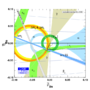eps png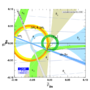eps png

 There is a specific correlation between sin2β and BR(B→τν), regarding the prediction from the global fit without using these measurements. The cross corresponds to the experimental value with 1 sigma errors.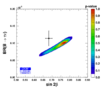eps png The constraint can also be seen from the point of view of lattice inputs, with the predictions on the decay constant fBd and fBd Sqrt[BBd]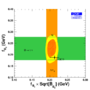eps png

 Constraints in the (|Vud|,|Vus|) plane. The indirect constraints (coming from b transitions) are related to |Vud| and |Vus| through unitarity. The red hashed region of the global combination corresponds to 68% CL.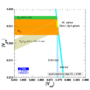eps png

 Constraints in the (|Vcd|,|Vcs|) plane. The indirect constraints (combing from b and s transitions) are related to |Vcd| and |Vcs| through unitarity. The direct constraints combine leptonic and semileptonic D and Ds decays as well as information from neutrino-nucleaon scattering and W → cs decays. The red hashed region of the global combination corresponds to 68% CL.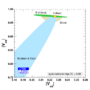eps png Constraints in the (|Vcd|,|Vcs|) plane where direct constraints involve only leptonic D and Ds decays with our inputs for lattice averages fD=249.2 ± 1.2 ± 4.5 MeV and fDs/fD=1.185 ± 0.005 ± 0.010.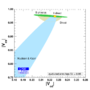eps png Constraints in the (|Vcd|,|Vcs|) plane where direct constraints involve only semileptonic D and Ds decays with our inputs for lattice averages FD → π(0)=0.666 ± 0.017 ± 0.048 and FD → K (0)=0.747 ± 0.010 ± 0.034.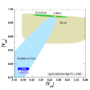eps png Constraints in the (|Vcd|,|Vcs|) plane where direct constraints involve only information from neutrino-nucleaon scattering and W→ cs decays (no lattice input).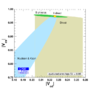eps png

 Constraints on γ/ϕ3 from world average D(*)K(*) decays (GLW+ADS) and Dalitz analyses (GGSZ): γ[combined] = 70.0+7.7-9.0°, compared to the prediction from the global CKM fit (not including these measurements): γ[fit] = 66.4+1.2-3.3°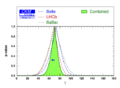eps png Constraints on γ/ϕ3 from all measurements on D(*)K(*) decays (GLW+ADS) and Dalitz analyses (GGSZ) (methods shown separately): γ[combined] = 70.0+7.7-9.0°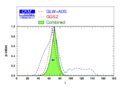eps png Constraints on γ/ϕ3 from BaBar measurements on D(*)K(*) decays (GLW+ADS) and Dalitz analyses (GGSZ) (methods shown separately): γ[BaBar] = 72+17-19°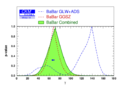eps png Constraints on γ/ϕ3 from Belle measurements on D(*)K(*) decays (GLW+ADS) and Dalitz analyses (GGSZ) (methods shown separately): γBelle] = 73+13-15°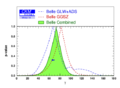eps png Constraints on γ/ϕ3 from LHCb measurements on D(*)K(*) decays (GLW+ADS) and Dalitz analyses (GGSZ) (methods shown separately):: γ[LHCb] = 68+12-15°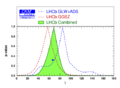eps png

 Constraint on the ratio of interfering amplitudes rB of the decay B → DK from world average D(*)K(*) decays (GLW+ADS) and Dalitz analyses (GGSZ): rB(DK) = 0.0972+0.0063-0.0064 .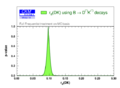eps png Constraint on the ratio of amplitudes rB of the decay B → D*K: rB(D*K) =0.120+0.018-0.020.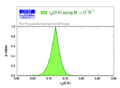eps png Constraint on the ratio of amplitudes rB of the decay B → DK*: rB(DK*) = 0.137+0.045-0.047.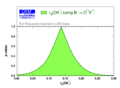eps png Constraint on the strong phase between the interfering amplitudes of the decay B → DK from world average D(*)K(*) decays (GLW+ADS) and Dalitz analyses (GGSZ): δB(DK) = 121.1+8.2-9.3°.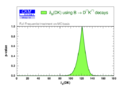eps png Constraint on the strong phase between the interfering amplitudes of the decay B → D*K: δB(D*K) = -50+13-15°.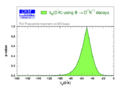eps png Constraint on the strong phase between the interfering amplitudes of the decay B → DK*: δB(DK*) = 107+35-42°.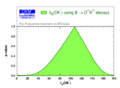eps png